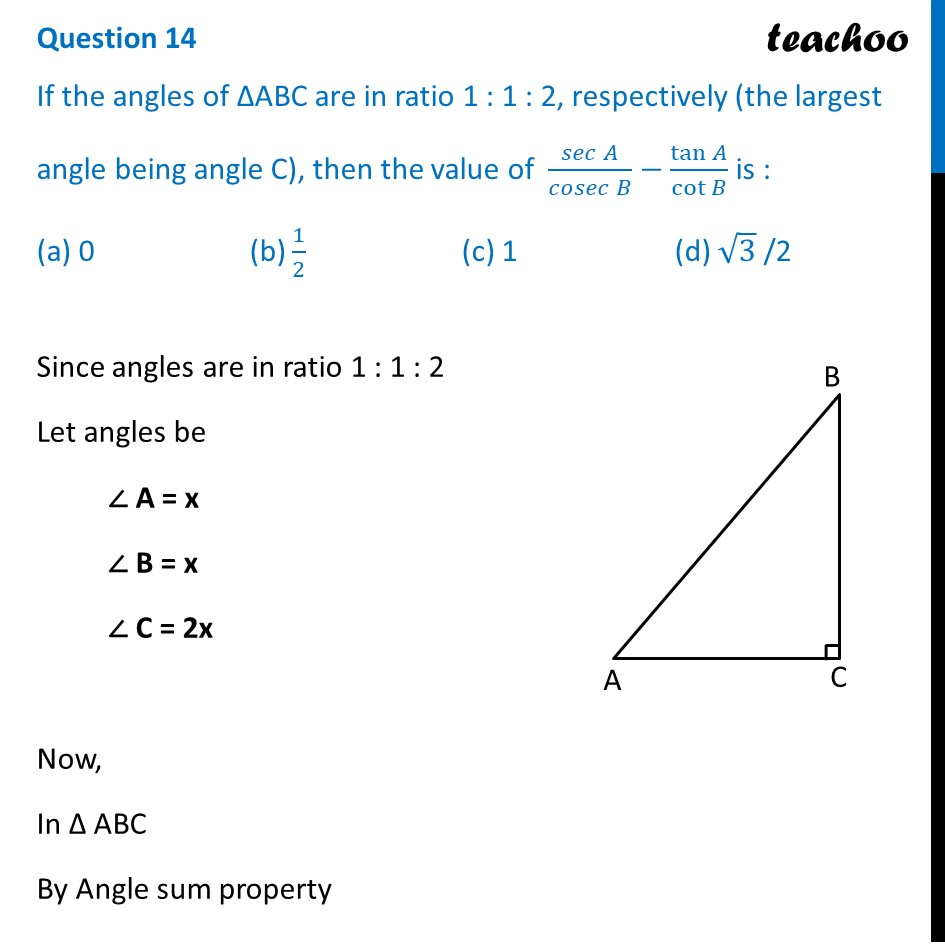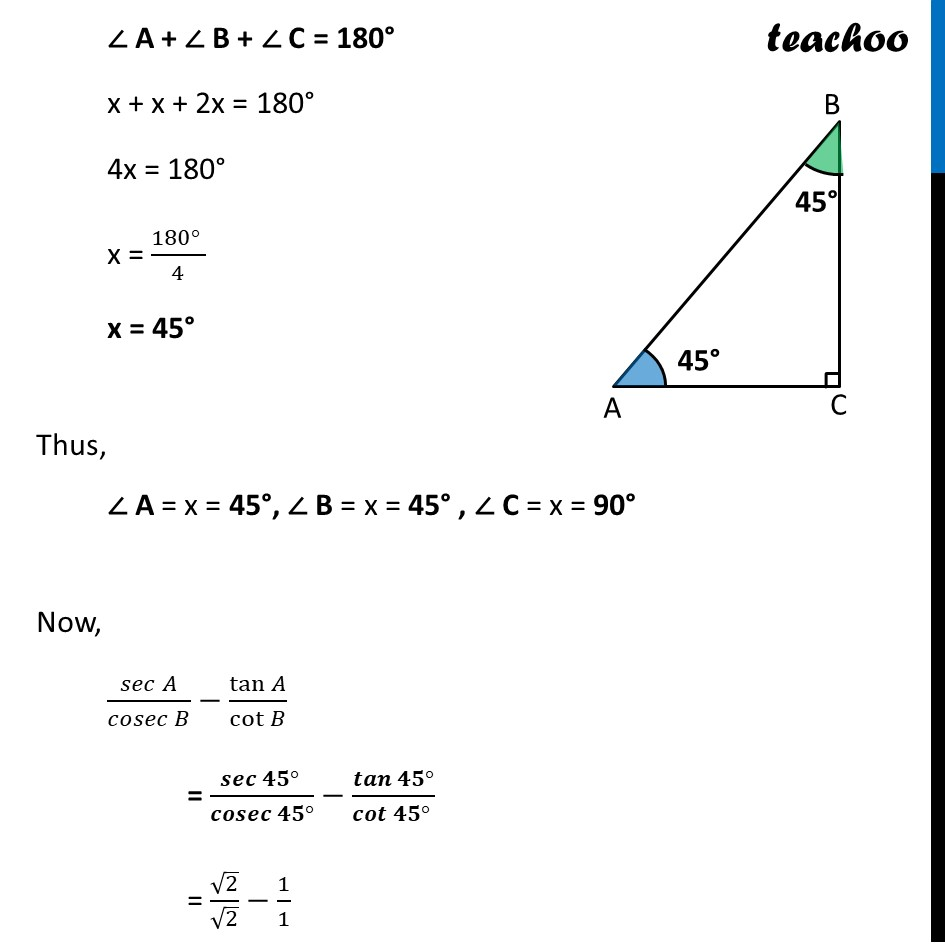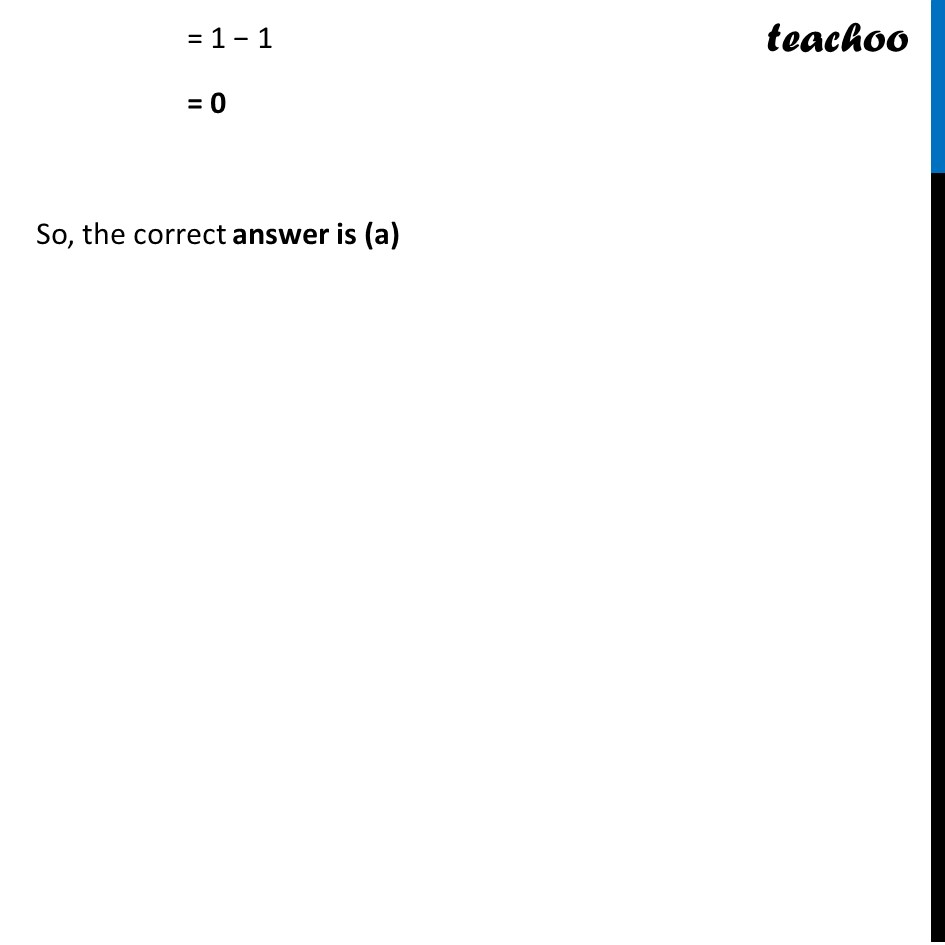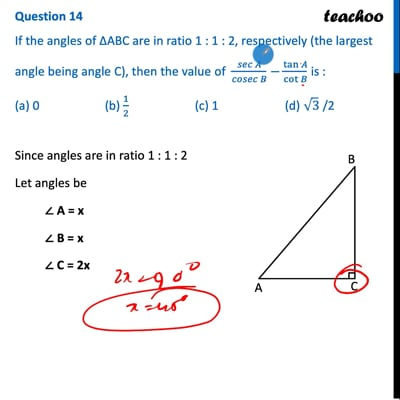CBSE Class 10 Sample Paper for 2022 Boards - Maths Standard [MCQ]

Class 10
Solutions of Sample Papers for Class 10 Boards

## (a) 0   (b) 1/2  (c) 1   (d) √3  /2This video is only available for Teachoo black users

Introducing your new favourite teacher - Teachoo Black, at only ₹83 per month

### Transcript

Question 14 If the angles of ∆ABC are in ratio 1 : 1 : 2, respectively (the largest angle being angle C), then the value of (𝑠𝑒𝑐 𝐴)/(𝑐𝑜𝑠𝑒𝑐 𝐵)−tan⁡𝐴/cot⁡𝐵 is : (a) 0 (b) 1/2 (c) 1 (d) √3 /2 Since angles are in ratio 1 : 1 : 2 Let angles be ∠ A = x ∠ B = x ∠ C = 2x Now, In Δ ABC By Angle sum property ∠ A + ∠ B + ∠ C = 180° x + x + 2x = 180° 4x = 180° x = (180° )/4 x = 45° Thus, ∠ A = x = 45°, ∠ B = x = 45° , ∠ C = x = 90° Now, (𝑠𝑒𝑐 𝐴)/(𝑐𝑜𝑠𝑒𝑐 𝐵)−tan⁡𝐴/cot⁡𝐵 = (𝒔𝒆𝒄 𝟒𝟓° )/(𝒄𝒐𝒔𝒆𝒄 𝟒𝟓°)−𝒕𝒂𝒏⁡〖𝟒𝟓°〗/𝒄𝒐𝒕⁡〖𝟒𝟓° 〗 = √2/√2−1/1 = 1 − 1 = 0 So, the correct answer is (a)